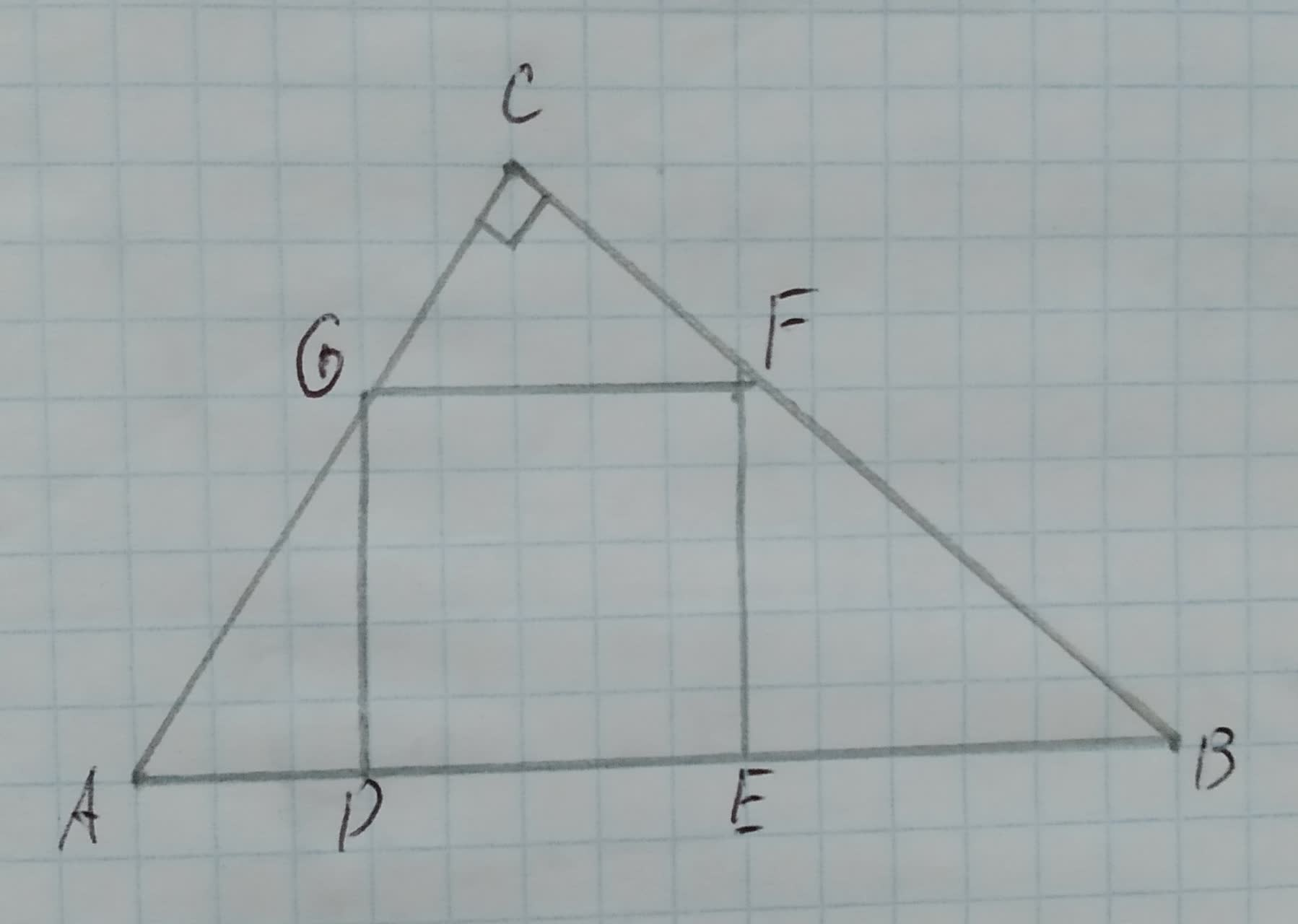# Proving Similarity in the figure DEFG is a square. Prove the following: AD*EB=DG*FE Given: The given figure is, 12210202691.jpgsibuzwaW 2020-11-29 Answered
Proving Similarity in the figure DEFG is a square. Prove the following:
Given:
The given figure is,You can still ask an expert for help

• Questions are typically answered in as fast as 30 minutes

Solve your problem for the price of one coffee

• Math expert for every subject
• Pay only if we can solve itBella
Approach:
The lengths of the corresponding sides in the triangles are proportional.
Calculation:
Since, $\mathrm{△}ADG\sim \mathrm{△}FEB$.
Thus, the lengths of their corresponding sides are proportional.
$\frac{AD}{FE}=\frac{DG}{EB}$
AD * EB = DG * FE
Therefore, it is proved that AD * EB = DG * FE.
Conclusion:
Hence, it is proved that AD * EB = DG * FE.